Courses

# RD Sharma Solutions - Ex-1.4 Integers, Class 7, Math Class 7 Notes | EduRev

## Class 7: RD Sharma Solutions - Ex-1.4 Integers, Class 7, Math Class 7 Notes | EduRev

The document RD Sharma Solutions - Ex-1.4 Integers, Class 7, Math Class 7 Notes | EduRev is a part of the Class 7 Course RD Sharma Solutions for Class 7 Mathematics.
All you need of Class 7 at this link: Class 7

#### QUESTION 1:

Simplify each of the following:
3 − (5 − 6 ÷ 3)

On applying the BODMAS rule, we get:
3 − (5 − 6 ÷ 3)
= 3 − (5 − 2)     (On performing division)
= 3 − 3              (On performing subtraction)
= 0

#### QUESTION 2:

Simplify each of the following:
−25 + 14 ÷ (5 − 3)

On applying the BODMAS rule, we get:
−25 + 14 ÷ (5 − 3)
= −25 + 14 ÷ 2       (On simplifying brackets)
= −25 + 7               (On performing division)
= −18

#### QUESTION 3:

Simplify each of the following: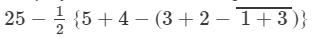On applying the BODMAS rule, we get: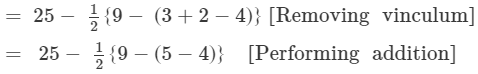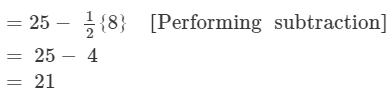#### QUESTION 4:

Simplify each of the following: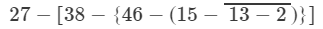On applying the BODMAS rule, we get:
27 - [38 - {46 - (15 -11)}]    (On simplifying vinculum)
= 27 - [38- {46 - 4}]              (On simplifying parentheses)
= 27 - [38 - 42]                       (On simplifying braces)
= 27 - (-4) = 31

#### QUESTION 5:

Simplify each of the following:
36[18{14(154 ÷2×2)}]36-18-{14-(15-4 ÷2×2)}

On applying the BODMAS rule, we get:
36 - [18 - { 14 - (15 - 4 ÷ 2 × 2)}]
= 36 − [18 − {14 − (15 − 2 × 2)}]                (On performing division)
= 36 - [18 - {14 - (15 - 4)}]                   (On performing multiplication)
= 36 - [18 - {14 - 11}]                            (On simplifying parentheses)
= 36 - [18 - 3]                                          (On simplifying braces)
= 36 - 15
= 21

#### QUESTION 6:

Simplify each of the following:
45[38{60÷3(69 ÷3)÷3}]45-38-{60÷3-(6-9 ÷3)÷3}

On applying the BODMAS rule, we get:

45 - [38 - { 60 ÷  3 - (6 - 9 ÷ 3) ÷ 3}]
=  45 - [38 - {60 ÷ 3 - (6 - 3) ÷ 3}]           (On performing division)
=  45 - [38 - {60 ÷ 3 - 3 ÷ 3}]                    (On simplifying parentheses)
=  45 - [38 - {60 ÷ 3 - 1}]                          (On performing division)
=  45 - [38 - {20 - 1}]                                (On performing division)
=  45 - [38 - 19]                                          (On performing subtraction)
=  45 - 19
= 26

#### QUESTION 7:

Simplify each of the following: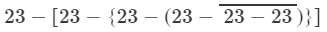On applying the BODMAS rule, we get:= 23 - [23 - {23 - (23 - 0}]            (On simplifying vinculum)
= 23 - [23 - {23 - 23}]                    (On simplifying parentheses)
= 23 - [23 - 0]                                  (On simplifying braces)
= 23 - 23 = 0

#### QUESTION 8:

Simplify each of the following: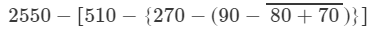On applying the BODMAS rule, we get:= 2550 - [510 - {270 - (90 - 150)}]        (On simplifying vinculum)
= 2550 - [510 - { 270 - (- 60)}]              (On simplifying parentheses)
= 2550 - [510 - 330]                                 (On simplifying braces)
= 2550 - 180
= 2370

#### Question 9:

Simplify each of the following: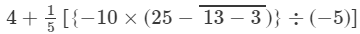On applying the BODMAS rule, we get: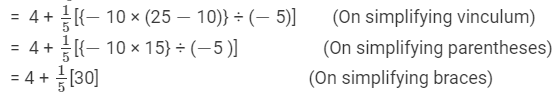= 4 + 6
= 10

####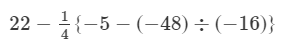On applying the BODMAS rule, we get: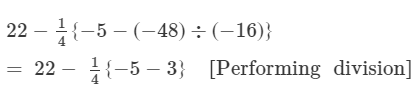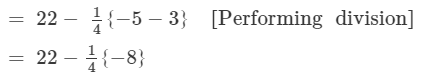=22(2)  [Removing braces]

= 22+2 = 24

#### Question 11:

Simplify each of the following: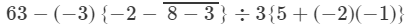#### nswer 11:

On applying the BODMAS rule, we get:=  63 - (- 3) {- 2 - 5} ÷ 3 {5 + 2}       (On simplifying vinculum)
= 63 - (- 3) (- 7 ) ÷ 3 × 7                     (On simplifying braces)
= 63 - (21÷2121÷21)
= 63 - 1
= 62

#### Question 12:

Simplify each of the following:
[29(2){6(73)}]÷[3×{5+(3)×(2)}]

On applying the BODMAS rule, we get:
[29 - (- 2) {6 - (7 - 3)}] ÷ [3 × { - 3) × (- 2)}]
= [29 - (- 2) {6 - 4}] ÷ [3 × { 5 + 6}]                       (On simplifying parentheses)
= [29 - (- 2) (2)] ÷ [3 × 11]                                        (On performing subtraction and addition)
= [29 + 4] ÷÷ 33                                                           (On performing multiplication)
= 33 ÷÷ 33
= 1

#### Question 13:

Using brackets, write a mathematical expression for each of the following:
(i) Nine multiplied by the sum of two and five.
(ii) Twelve divided by the sum of one and three.
(iii) Twenty divided by the difference of seven and two.
(iv) Eight subtracted from the product of two and three.
(v) Forty divided by one more than the sum of nine and ten.
(vi) Two multiplied by one less than the difference of nineteen and six.

(i) 9 (2 + 5)
(ii) 12 ÷ (1 + 3)
(iii) 20 ÷ (7 - 2)
(iv) (2 × 3 ) - 8
(v) 40 ÷ {(9 + 10) + 1}
(vi) 2 × {(19 - 6) - 1}

The document RD Sharma Solutions - Ex-1.4 Integers, Class 7, Math Class 7 Notes | EduRev is a part of the Class 7 Course RD Sharma Solutions for Class 7 Mathematics.
All you need of Class 7 at this link: Class 7Use Code STAYHOME200 and get INR 200 additional OFF Use Coupon Code
All Tests, Videos & Notes of Class 7: Class 7

### Top Courses for Class 7## RD Sharma Solutions for Class 7 Mathematics

97 docs

### Top Courses for Class 7Track your progress, build streaks, highlight & save important lessons and more!

,

,

,

,

,

,

,

,

,

,

,

,

,

,

,

,

,

,

,

,

,

,

,

,

,

,

,

;